JEE  >  Matrix-Match & Integer Answer Type Questions: The p-Block Elements | JEE Advanced

# Matrix-Match & Integer Answer Type Questions: The p-Block Elements | JEE Advanced - Notes | Study Chemistry 35 Years JEE Main & Advanced Past year Papers - JEE

 1 Crore+ students have signed up on EduRev. Have you?

Match the Following

DIRECTIONS (Q. 1 to 3) : Each question contains statements given in two columns, which have to be matched. The statements in Column-I are labelled A, B, C and D, while the statements in Column-II are labelled p, q, r, s and t. Any given statement in Column-I can have correct matching with ONE OR MORE statement(s) in Column-II. The appropriate bubbles corresponding to the answers to these questions have to be darkened as illustrated in the following example :

If the correct matches are A-p, s and t; B-q and r; C-p and q; and D-s then the correct darkening of bubbles will look like the given.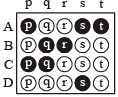Q.1. Match gases under specified conditions listed in Column I with their properties/laws in Column II. Indicate your answer by darkening the appropriate bubbles of the 4 × 4 matrix given in the ORS.

Column I                                        Column II

(A) Explosive                                      (p) NaN3

(B) Artificial gem                                (q) Fe3O4

(C) Self reduction                               (r) Cu

(D) Magnetic material                        (s) Al2O3

(t) Pb(N3)2

(u) Fe2O3

(v) Cu

(w) SiC

Ans. (A)-t; (B)-s; (C)-v; (D)-u

Solution. (A)-(t),   Pb(N3)2 is an explosive
(B)-(s),  Al2O3 is used to prepare artificial gun
(C)-(v),  Extraction of copper involves self-reduction process.
(D)-(u),  Fe2O3 is a magnetic material.

Q.2. Match the following :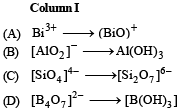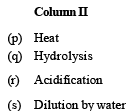Ans. (A)-q; (B)-s; (C)-p; (D)-r

Solution. (A)-(q), Bi3+ hydrolyses to yield BiO+ ion
(B)-(s), AlO2 on dilution yields a white ppt. of Al(OH)3
(C)-(p), When heated (SiO4)4– changes to (Si2O7)6–
(D)-(r), When acidified (B4O7)2– gives B(OH)3 (or H3BO3)

Q.3. Match each of the diatomic molecules in Column I with its property/properties in Column II.

Column I                                              Column II

(A) B2                                      (p) Paramagnetic

(B) N2                                     (q) Undergoes oxidation

(C) O2                                  (r) Undergoes reduction

(D) O2                                    (s) Bond order > 2

(t) Mixing of  's' and 'p' orbital

Ans. (A)-p, r, t; (B)-s, t ; (C)-p, q, r ; (D)-p, r, s

Solution.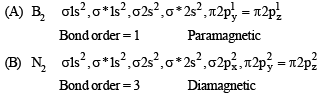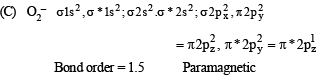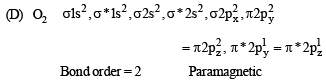DIRECTIONS (for Q. 4) : Following question has matching lists. The codes for the lists have choices (a), (b), (c) and (d) out of which ONLY ONE is correct.

Q.4. The unbalanced chemical reactions given in List I show missing reagent or condition (?) which are provided in List II. Match List I with List II and select the correct answer using the code given below the lists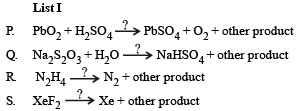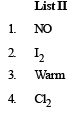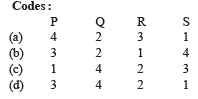Ans. (d)

Solution.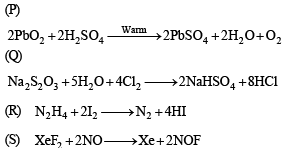Integer Value Correct Type

Q.1. The coordination number of Al in the crystalline state of AlCl3 is                  (2009)

Ans. 6

Solution. Coordination number of Al is 6. It exists in ccp lattice with 6 coordinate layer structure.

Q.2. The value of n in the molecular formula BenAl2Si6O18 is              (2010)

Ans. 3

Solution. Total cationic charge = Total anionic charge

2n + 6 + 24 = 36 ⇒ n = 3

Q.3. Reaction of Br2 with Na2CO3 in aqueous solution gives sodium bromide and sodium bromate with evolution of CO2 gas. The number of sodium bromide molecules involved in the balanced chemical equation is           (2011)

Ans. 5

Solution. 3Br2 + 3Na2CO 3 → 5NaBr + NaBrO3+ 3CO2

Q.4. Among the following, the number of compounds than can react with PCl5 to give POCl3 is  (2011)

O2, CO2, SO2, H2O, H2SO4, P4O10

Ans. 4

Solution. PCl5 + SO2 → POCl3 + SOCl2
PCl5 + H2O →POCl3 + 2HCl
PCl5 + H2SO4 → POCl3 + SO2Cl2 + 2HCl
6PCl5 + P4O10 → 10POCl3

Q.5. The total number of lone pairs of electrons in N2O3 is                (JEE Adv. 2015)

Ans. 8

Solution.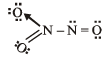Number of lone pairs = 8

Q.6. Three moles of B2H6 are completely reacted with methanol. The number of moles of boron containing product formed is    (JEE Adv. 2015)

Ans. 6

Solution. 3B2H6 + 18CH3OH → 6B(OCH3)3 + 18H2

The document Matrix-Match & Integer Answer Type Questions: The p-Block Elements | JEE Advanced - Notes | Study Chemistry 35 Years JEE Main & Advanced Past year Papers - JEE is a part of the JEE Course Chemistry 35 Years JEE Main & Advanced Past year Papers.
All you need of JEE at this link: JEE

## Chemistry 35 Years JEE Main & Advanced Past year Papers

142 docs|66 tests
 Use Code STAYHOME200 and get INR 200 additional OFF

## Chemistry 35 Years JEE Main & Advanced Past year Papers

142 docs|66 tests

Track your progress, build streaks, highlight & save important lessons and more!

,

,

,

,

,

,

,

,

,

,

,

,

,

,

,

,

,

,

,

,

,

;